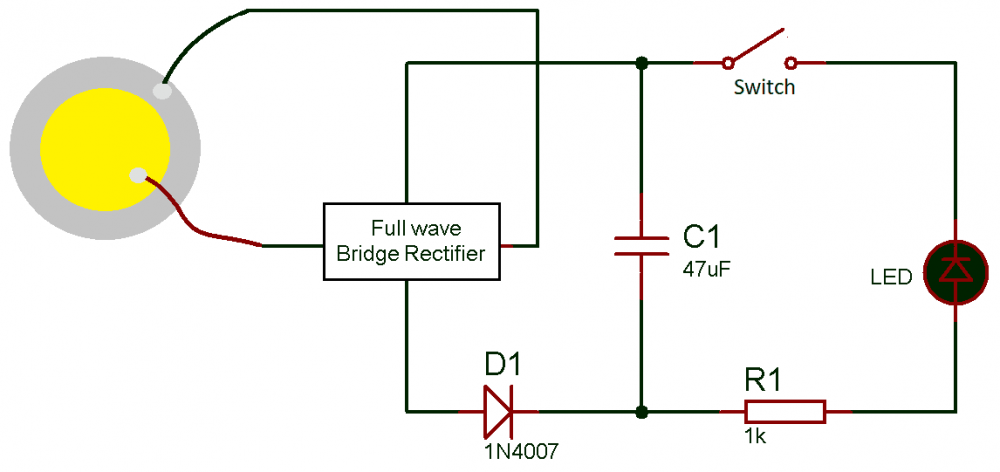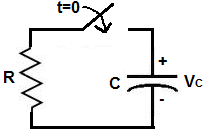# Capacitor Discharging Time

6 posts / 0 newOffline

Joined: Sep 24, 2018

Replies: 0
Capacitor Discharging Time
September 24, 2018 - 6:24pm

Hi i have designed a circuit for electricity generation using Piezoelectric crystal. As you can see in the below attached circuit diagram, the working concept is like that, the capacitor first charges by pressing the piezo crystal. And when the switch is pressed the capacitor transferred all its stored energy into the LED, and we get a flash of LED. it seems that the ON time of LED depends on the value of capacitor..  so can anyone explain how to calculate the discharging time of the capacitor so i can increase the ON time of the LED..Offline

Joined: May 19, 2015

Replies: 213

Calculating Energy stored in Capacitor and time taken:

The amount of energy that can be stored in a capacitor can be calculated by using the formulae below

E = CV/ 2

where,

E = Energy stored in capacitor in joules

C = Capacitance of the capacitor in farads

V = Voltage applied across the capacitor in Volts

In your case for a 47uF capacitor assuming your crystal produces 3V constantly (which is not possible) the energy stored will be

E = 0.21mJ

The time taken for the capacitor to charge will be

τ = RC

τ = Time constant in seconds

R = Resistance in ohms

Calculating the discharge time and energy:

Once we know how much energy is stored in the capacitor we can assume it to be a voltage source (battery) and then based on the current consumed by the load we can calculate the discharge time of the capacitor.

Remember these calculations will only give a rough figure and will not be accurate since we are not considering the nature of the circuit and the impedance associated with capacitorOffline

Joined: Nov 21, 2019

Replies: 36

To calculate the time constant of a capacitor, the formula is τ=RC. This value yields the time that it takes a capacitor to discharge to 63% of the voltage that is charging it up. After 5 time constants, the capacitor will discharge to almost 0% of all its voltage.

The Capacitor Discharge Calculator calculates the voltage that a capacitor with a a capacitance, of C, and a resistor, R, in series with it, will discharge to after time, t, has elapsed. You can use this calculator to calculate the voltage that the capacitor will have discharged after a time period, of t, has elapsed.

In order to calculate the voltage across the capacitor, we must know the initial voltage, V0, across the capacitor before the discharging begins, the capacitance, C, of the capacitor, the resistor, R, in series with the capacitor, and the amount of time that has elasped since the discharging began. Once we know these, we can calculate the voltage across the capacitor using the formula shown above.The 3 variables which affect how the inital voltage discharges is time, t, the resistance of the resistor, R, and the capacitance of the capacitor, C.

The greater the amount of time has elapsed, the more the capacitor will discharge. The less time that has elapsed, the less time the capacitor has to discharge. Thus, the larger the t value, the smaller VC.Offline

Joined: Nov 07, 2019

Replies: 124

for all extensive purposes, the capacitor will be discharged of nearly all its voltage. A capacitor never discharges fully to zero volts, but does get very close.

Everything we have done has assumed that things are in steady state: all fields are constant; charges are either nailed in place or else are flowing uniformly. In reality, such a situation tends to be the exception rather than the rule. We now start to think about things that vary in time. Suppose we have a capacitor C charged up until the potential difference between its plates is V0; the charge separation is Q0 = CV0. We put this capacitor into a circuit with a resistor R and a switch s.Before thinking about this with equations, let’s think about what happens here physically. The instant that the switch is closed, there is a potential difference of V0 across the resistor. This drives a current to flow. Now, this current can only come from the charge separation on the plates of the capacitor: the excess charges on one plate flow off and neutralize the deficit of charges on the other plate. The flow of current thus serves to reduce the amount of charge on the capacitor; by Q = CV , this must reduce the voltage across the capacitor. The potential difference which drives currents thus becomes smaller, and so the current flow should reduce. We expect to see a flow of current that starts out big and gradually drops off.Offline

Joined: Aug 22, 2019

Replies: 125

Capacitor discharge (voltage decay)  V = Voe-(t/RC), where Vo is the initial voltage applied to the capacitor.

E=CV2/2

τ=RC

Where: V= applied voltage to the capacitor (volts)

R= resistance (ohms)

τ= time constant (seconds)Offline

Joined: Nov 07, 2019

Replies: 124

A fully charged capacitor discharges to 63% of its voltage after one time period. After 5 time periods, a capacitor discharges up to near 0% of all the voltage that it once had. Therefore, it is safe to say that the time it takes for a capacitor to discharge is 5 time constants.

To calculate the time constant of a capacitor, the formula is τ=RC. This value yields the time that it takes a capacitor to discharge to 63% of the voltage that is charging it up. After 5 time constants, the capacitor will discharge to almost 0% of all its voltage.